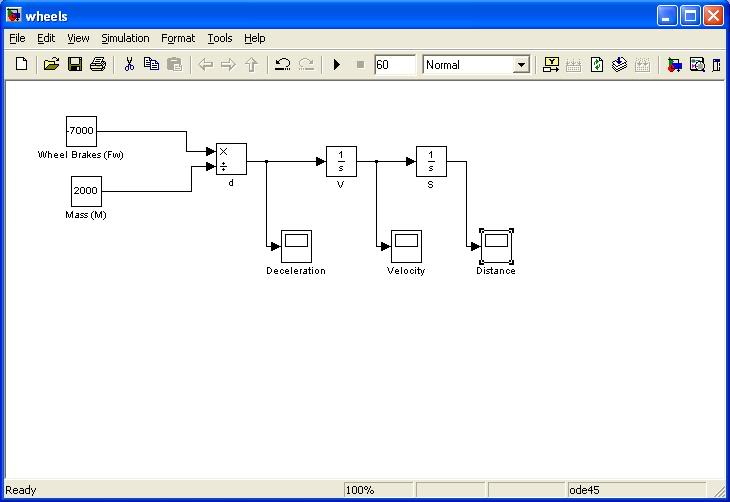[Solved] A little matlab help needed

Homework Statement

right, im modelling the deceleration of a vehicle in simulink

the vehicle uses wheel brakes, and a parachute to decellerate from Vmax to zero

I have constructed a model which models the constant force of the brakes to decelerate the vehicle to stop

I need to amend the model, so that the velocity dependant drag force produced by the parachute, and the drag of the vehicle itself are taken into account.

I am ignoring any turbulence produced

Homework Equations

Fd=0.5CdpAu^2

where
Cd is the drag coefficient of the parachute or car
p is the density
A is the frontal area of the parachute or car
u is the velocity

The Attempt at a Solution

so far as said above, i have the deceleration from the wheel brakes, shown here:what type of block do i need to use to add the variable drag force to this system?

Many Thanks
Ed